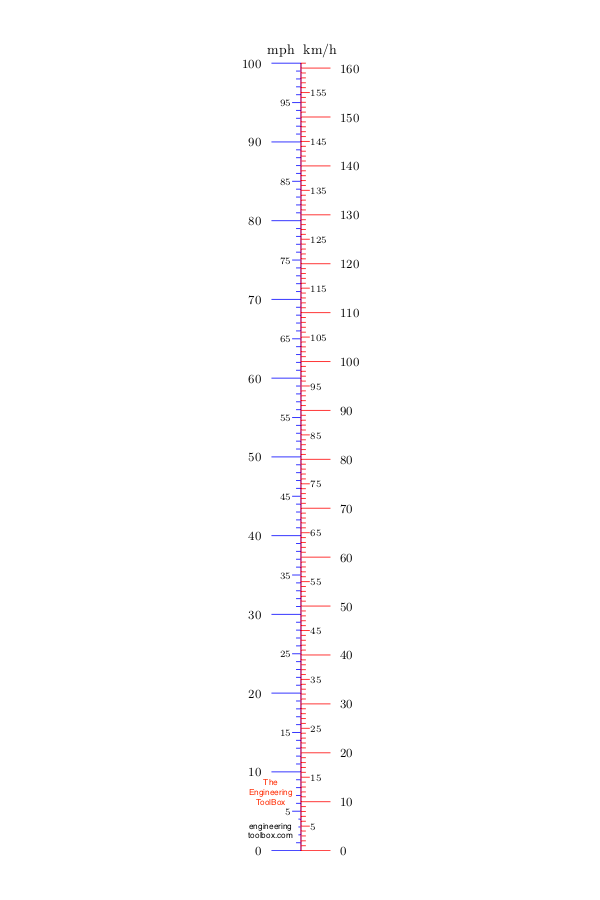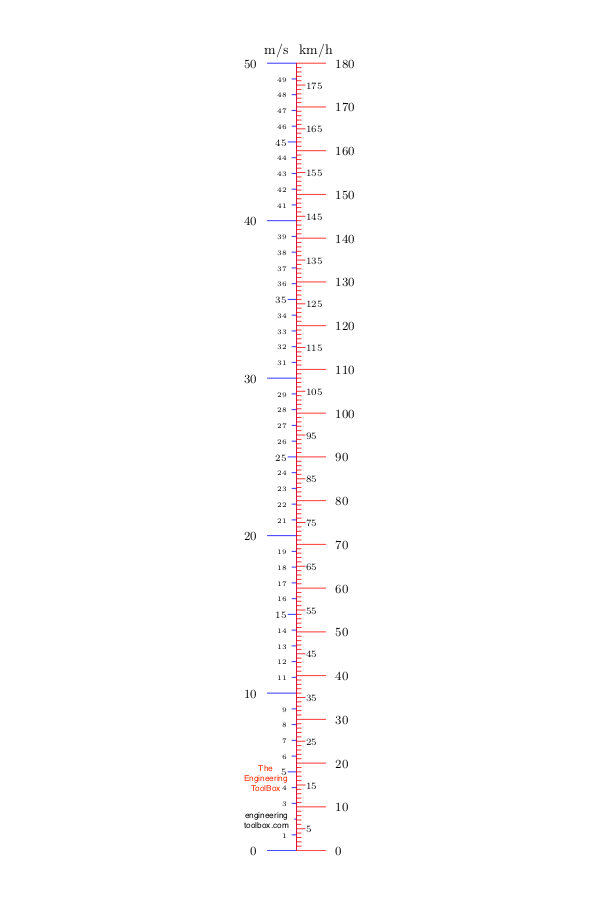Engineering ToolBox - Resources, Tools and Basic Information for Engineering and Design of Technical Applications!

# Velocity Units - Online Converter

## Convert between common velocity and speed units.

### Online Velocity Units Calculator

The calculator below can be used to convert between common velocity and speed units:

Value

m/s

km/h

ft/min

ft/s

yards/min

mph

knots

### Related Mobile Apps from The Engineering ToolBox- free apps for offline use on mobile devices.

### Velocity Units Converting Table

The table below can be used to convert between common velocity and speed units:

 Multiply by Convert from Convert to m/s km/h ft/min ft/s yards/min mph knots m/s 1 3.6 196.9 3.28 65.6 2.24 1.94 km/h 0.278 1 54.68 0.91 18.23 0.62 0.54 ft/min 5.08 10-3 1.83 10-2 1 1.67 10-2 0.33 1.14 10-2 9.87 10-3 ft/s 0.305 1.097 60 1 20 0.682 0.592 yards/min 1.52 10-2 5.49 10-2 3 0.05 1 3.41 10-2 2.96 10-2 mph 0.45 1.609 88 1.47 29.33 1 0.869 knots 0.51 1.85 101.3 1.69 33.76 1.15 1

### Some other common Velocity Units

• 1 metre per second = 6000 centimetre per minute
• 1 metre per second = 100 centimetre per second
• 1 metre per second = 11811 feet per hour
• 1 metre per second = 2362.2 inches per minute
• 1 metre per second = 39.37 inches per second
• 1 metre per second = 1.942615 knots
• 1 metre per second = 3600 metre per hour
• 1 metre per second = 60 metre per minute
• 1 metre per second = 0.0373 miles per minute
• 1 metre per second = 0.00062 miles per second
• 1 metre per second = 3937 yards per hour

### mph to km/h Converter### m/s to km/h Converter## Related Topics

• ### Basics

The SI-system, unit converters, physical constants, drawing scales and more.

## Related Documents

• ### Acceleration Units Converter

Converting between units of acceleration.
• ### Acceleration vs. Velocity Equations

Useful equations related to acceleration, average velocity, final velocity and distance traveled.
• ### Average Velocity

Distance traveled vs. time used.
• ### Unit Converter with commonly used Units

Common converting units for Acceleration, Area, Density, Energy, Energy per unit mass, Force, Heat flow rate, Heat flux, Heat generation per unit volume and many more.
• ### Unit Factor Method

Convert between units with the unit factor or factor-label method
• ### Velocity Units Converter

Convert between speed and velocity units like m/s, km/h, knots, mph and ft/s.
• ### Volume Flow - Online Unit Converter

Convert between volume flow units like gpm, liter/sec, cfm, m3/h.

## Engineering ToolBox - SketchUp Extension - Online 3D modeling!

Add standard and customized parametric components - like flange beams, lumbers, piping, stairs and more - to your Sketchup model with the Engineering ToolBox - SketchUp Extension - enabled for use with older versions of the amazing SketchUp Make and the newer "up to date" SketchUp Pro . Add the Engineering ToolBox extension to your SketchUp Make/Pro from the Extension Warehouse !

We don't collect information from our users. More about

## Citation

• The Engineering ToolBox (2007). Velocity Units - Online Converter. [online] Available at: https://www.engineeringtoolbox.com/velocity-units-converter-d_1035.html [Accessed Day Month Year].

Modify the access date according your visit.

9.19.12